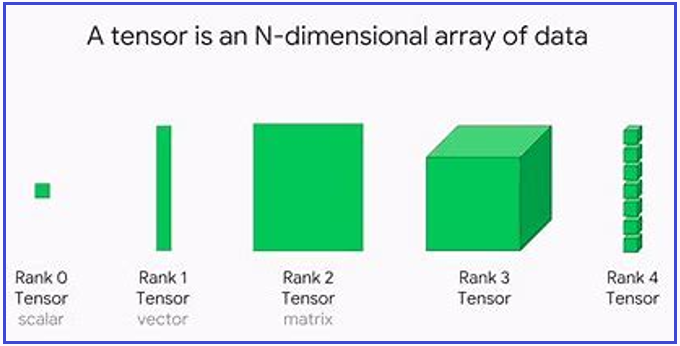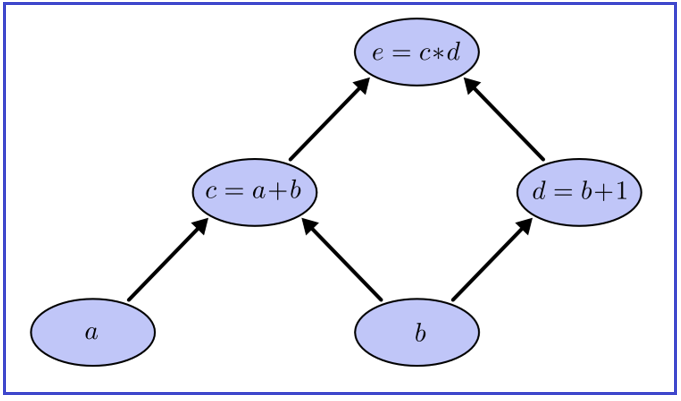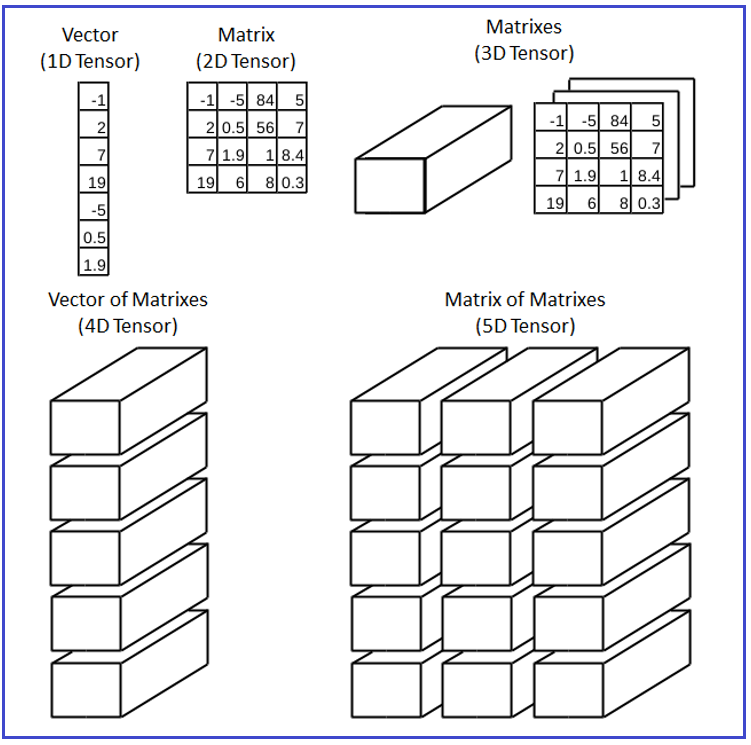# Deep Learning with Tensorflow

## Deep Learning with Tensorflow

In this article, I am going to discuss Deep Learning with Tensorflow. Please read our previous article where we give a brief Introduction to Deep Learning and AI.

##### What is Tensorflow?

TensorFlow, created by Google, is one of the most well-known and commonly used libraries for creating and deploying Machine Learning and other algorithms with a lot of complex mathematical operations.

In order to establish an ecosystem that offers a collection of workflows for developing and training models, and for integrating machine learning in virtually all applications, Google launched TensorFlow. In actuality, TensorFlow is used by all of us frequently without our knowledge: When you use Google Photos or Google Voice, you are indirectly employing TensorFlow models since they are effective at performing perceptual tasks and run-on enormous clusters of Google hardware.

TensorFlow = Tensor + Flow

Tensors and an interconnected computational graph with edges constitute TensorFlow’s essential elements.

TensorFlow is essentially a software package that uses data flow graphs to compute numerically in the following ways:

1. The graph’s nodes correspond to mathematical operations.
2. The multidimensional data arrays (referred to as tensors) transferred between the nodes of the graph are represented by its edges. (Note that the primary unit of data in TensorFlow is the tensor.)

With only one API, you can use this adaptable architecture to deliver computing to one or more CPUs or GPUs on a desktop, server, or mobile device!

Before using Tensorflow in your program, first, you need to install it on your device. You can follow a few steps from this link to get the library installed. After successful installation, you need to import it first, and then, it is ready to be used.

import tensorflow as tf

##### Why Tensorflow?

Before understanding the reason behind the popularity of Tensorflow, let’s understand its major features.

##### Features of Tensorflow –
1. Model development is simple. TensorFlow features high-level APIs that make it simple to construct Machine Learning models using Neural Networks.
2. One can perform complex numerical computations. The huge size of the input dataset doesn’t make it difficult to perform mathematical calculations.
3. Both low-level and high-level machine learning APIs are abundant in TensorFlow. Python and C both have stable APIs available.
4. Simple model training and model construction using CPU and GPU are supported by TensorFlow. Both CPU and GPU computations can be performed, and they can also be compared.
5. Contains pre-trained models and datasets: TensorFlow comes with a tonne of pre-trained models and datasets thanks to Google.
6. On top of TensorFlow and Theano, Keras is a high-level TensorFlow API. Nowadays, Keras is a well-liked TensorFlow API that is frequently used.
7. TensorFlow is an open-source, cost-free platform that enables researchers and developers to create and use machine learning models.
##### Here are the reasons why Tensorflow is so popular.
1. With pre-trained models, data, and high-level APIs, TensorFlow simplified machine learning, making it simple for anybody to create ML models.
2. Typically, TensorFlow is mostly used by researchers and students to develop models and conduct research.
3. TensorFlow supports pre-trained models that may be used right away for production and experiment.
4. TensorFlow is used to make machine learning available as a service. The necessary model from the TensorFlow models can be used.
5. Many businesses use TensorFlow, including Google, Intel, DeepMind, Twitter, Uber, DropBox, AirBnB, and others. TensorFlow is used by more than 400 businesses.
##### Tensorflow as an Interface

TensorFlow has numerous APIs (Application Programming Interfaces). These can be divided into two main groups:

###### Low-Level API –
1. Total control over the programming
2. Recommended for researchers in machine learning
3. Gives the models a high degree of control
4. The low-level TensorFlow API is called TensorFlow Core
###### High-Level API –
1. Constructed atop TensorFlow Core
2. More ease of use and learning than TensorFlow Core
3. Simplify and standardize repetitive processes for consumers across numerous platforms.
4. A high-level API is one like tf.contrib.learn
##### Tensorflow as an Environment

The environment is an agent’s immediate surroundings or workspace. The environment changes as a result of the agent’s interactions with it. Even while TensorFlow offers contexts for some common tasks like CartPole, we occasionally get across situations where we have to create our own environments.

Environments in TF-Agents can be implemented using TensorFlow or Python. Although TensorFlow environments are more efficient and support natural parallelization, Python environments are typically simpler to construct, comprehend, and debug. The most typical process is to build an environment in Python and then utilize one of our wrappers to transform it into TensorFlow automatically.

##### Tensors

As its name suggests, TensorFlow is a framework for creating and using tensor-based computations. A tensor is a vector or matrix that has been expanded to potentially more dimensions. Tensors are internally represented by TensorFlow as n-dimensional arrays of fundamental data types. The data type of every element in the tensor is the same and is always known. It’s possible that only a portion of the shape—that is, the number of dimensions and sizes of each—is known. When the forms of their inputs are fully known, the majority of operations yield tensors with fully known shapes, but in rare circumstances, the shape of a tensor can only be determined when the graph is being executed.

This is a general explanation of how tensors work in mathematics.

A scalar is always 1×1 and has the lowest dimensionality. It can be compared to a 1×1 matrix or a 1×1 vector. It is followed by a vector with a scalar as each of its elements. A vector’s dimensions are simply Mx1 or 1xM matrices.

Then there are matrices, which are merely a collection of vectors. A matrix has the dimensions MxN. In other words, a matrix is a grouping of n vectors, each with m by 1 dimension. or m vectors, each with n by 1 dimension.

Tensors of ranks 0, 1, and 2 are what scalers, vectors, and matrices are, respectively. Simply said, tensors are an extension of the ideas we have covered thus far.

A tensor of rank 3 is a thing we haven’t seen. It is a KxMxN object since k, m, and n could be used to represent its dimensions. One way to conceptualize such an object is as a collection of matrices.##### Computation Graphs

a particular kind of graph that is suitable for displaying mathematical statements. In the case of deep learning models, this is comparable to descriptive language in that it offers a functional description of the necessary computation.

The computational graph, which is used to represent and evaluate mathematical statements, is often a directed graph. We can perform calculations for both – Forward propagation and Backward propagation with these.

A few important computational graphs terms are defined in the sections that follow –

1. A node in a network represents a variable. It could be a tensor, matrix, scalar, vector, or even another kind of variable.
2. An edge can be used to represent both a function argument and a data dependency. These resemble node pointers in structure.
3. An operation is a straightforward mathematical procedure on one or more variables. A specific set of operations are allowed. Multiple operations can be combined to represent functions that are more complicated than those in this collection.

Now let’s take a very simple example of this function –

e = (a+b)*(b+1)

Here we have two different operations (addition and multiplication) based on two inputs a and b. So, we will use inputs a and b as leaf nodes, and for every operation, a further node will be created. The direction of the arrows represents the order of computations to be made. This way computation graphs make it easier to perform complex calculations for forward and backward propagation.

Here’s what the computation graph for the above equation is going to look like.##### Installing Tensorflow

Python must be set up on your machine in order to install TensorFlow. The recommended Python version to start with when installing TensorFlow is 3.4 or later. The Tensorflow may be installed on the system using pip. The installation command is listed as follows:

pip install tensorflow

You can use this command for Tensorflow installation using Anaconda Prompt (if you have anaconda prompt installed).

##### Types of Tensors

The most typical tensor types are:

1. 1D tensors
2. 2D tensor
3. 3D tensors (used for time series)
4. 4D tensors (used for images)
5. 5D tensors (used for videos)##### Running TensorFlow Programs

Let’s have a look at how TensorFlow programs in general work –

###### Import Data

First, import the library to work on it. Data will be the basis for every machine learning algorithm we develop. In actual life, we will either develop data or use data from a different source. Because we often want to know what to expect, it can be preferable to rely on generated data. Furthermore, TensorFlow already includes well-known datasets like MNIST, CIFAR-10, etc.

import tensorflow

###### Normalize and transform data

Typically, the data does not conform to the dimension or type that our Tensorflow algorithms require. Before we can use the data, we must alter it. Additionally, most algorithms demand standardized data. The built-in functions of Tensorflow can normalize the data for you.

df = tensorflow.nn.batch_norm_with_global_normalization(…)

###### Set the parameters for the algorithm

Typically, we maintain a set of parameters for our algorithms throughout the process. This could be, for instance, the number of iterations, the learning rate, or other predetermined fixed parameters. Initializing these jointly is regarded as good practice so that the reader or user can quickly locate them.

###### Set up variables

We must specify to Tensorflow what it may and cannot modify. During optimization, Tensorflow will change the variables in order to reduce a loss function. We insert data through placeholders to do this. In order for Tensorflow to know what to expect, we must initialize both of these—variables and placeholders—with size and type.

###### Setting up the Model’s structure

We must define the model once we have the data, and initialized our variables, and placeholders. By creating a computational graph, this is accomplished. To get to our model predictions, we instruct Tensorflow on the operations that need to be performed on the variables and placeholders.

###### Initialize the Loss Function

We must be able to assess the result after the model has been defined. We declare the loss function at this point. The loss function is crucial because it indicates how far off from the actual values our forecasts are.

###### Model Training

Now that everything is set up, we can build an instance of our graph, feed data into the placeholders, and let Tensorflow adjust the variables to more accurately forecast the training data.

###### Model Evaluation

After the model has been created and trained, it should be assessed by seeing how well it performs on fresh data using a set of predetermined criteria.

###### Predict Results

It’s also crucial to understand how to generate forecasts based on brand-new, unforeseen facts. Once we’ve trained them, we can use all of our models for this.

##### Tensorflow Optimizers

The expanded class, or optimizers, includes extra data to train a particular model. Although the optimizer class is initialized with the specified parameters, it is crucial to keep in mind that no Tensor is required. The optimizers are used to increase efficiency and effectiveness when training a particular model. The fundamental TensorFlow optimizer is tensorflow.train.Optimizer

The following list of Tensorflow optimizers –

3. Momentum
4. Nesterov kinetics
7. RMSProp
10. SMORMS3

#### CPU vs GPU vs TPU

Let’s have a look at the features for each one of them and why they should be chosen for a certain AI application –

##### CPU –
###### Features –
1. has several low-latency cores with a focus on serial processing
2. able to do a number of operations at once
3. Due to its enormous memory capacity, it supports the largest model.
4. a great deal more adaptable and customizable for erratic calculations (e.g., small batches non MatMul computations)
5. prototypes that demand the most adaptability
###### When to Choose?
1. Basic models that don’t take a lot of time to train
2. Using minimal effective batch sizes while training tiny models
3. primarily created using modified TensorFlow techniques and written in C++
4. models with constrained system networking bandwidth or limited I/O
##### GPU –
###### Features –
1. Has a huge number of cores
2. Maximum output
3. Parallel processing expertise
4. Able to perform tens of thousands of processes simultaneously
###### Why Choose?
1. Models that require a GPU to handle multiple unique TensorFlow operations
2. Models excluded from the Cloud TPU
3. Models of medium or greater size and larger effective batch sizes
##### TPU –
###### Features –
1. Hardware Specific for Matrix Processing
2. A long latency (compared to CPU)
3. Outstanding Throughput
4. Use extreme parallelism when computing
5. High-performance for CNNs and huge batches (convolutional neural network)
###### When to Choose?
1. Training models primarily with matrix operations
2. Training models inside the main training loop without using customized TensorFlow methods
3. Models of training that take weeks or months to complete
4. Using extremely large effective batch sizes for training massive models